/

### Function Description

Returns the fast Fourier transform (‘FFT’) (if CarryOutForwardTransform is true) or the inverse FFT (if CarryOutForwardTransform is false) of an array ofcomplex numbers (i.e. input array has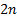term, the real part followed by the imaginary part), wheremust be an integral power of two.

If the input to the FFT is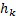(eachis a complex number) where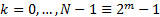then the output (of the forward transform) is another array of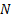complex numbers as follows: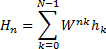where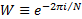. When inverting, a constant multiplier is applied to all terms to ensure that the result of applying the transform to the output of the forward transform and then applying the transform returns the original series.

The FFT calculates the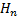using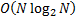rather than the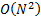that might appear to be needed given the above formula. This involves a very large speed up for large data analyses, e.g. analysing large pictures or other datasets.

Some writers use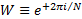, e.g. Press et al. (2007), and some writers multiply the expression with constants, e.g.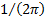. The use ofmeans that the end result is compatible with output from the Microsoft Excel Data Analysis Toolpack.

Contents | Prev | Next